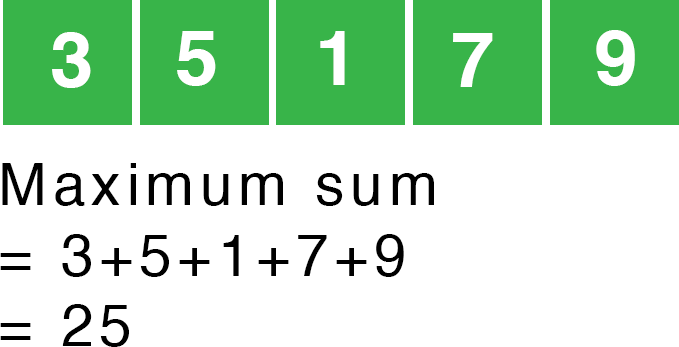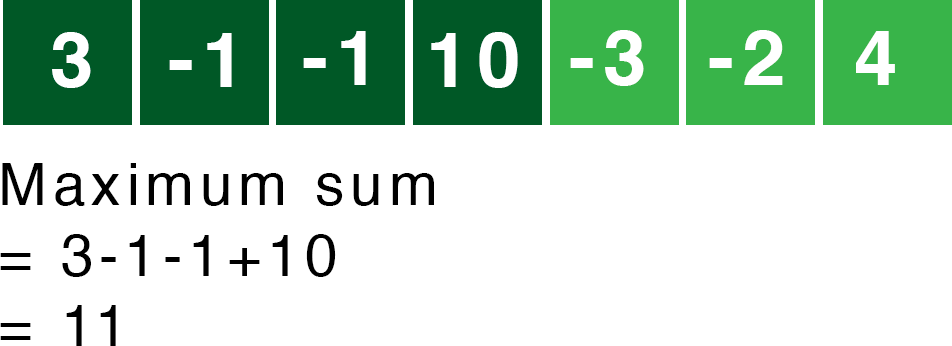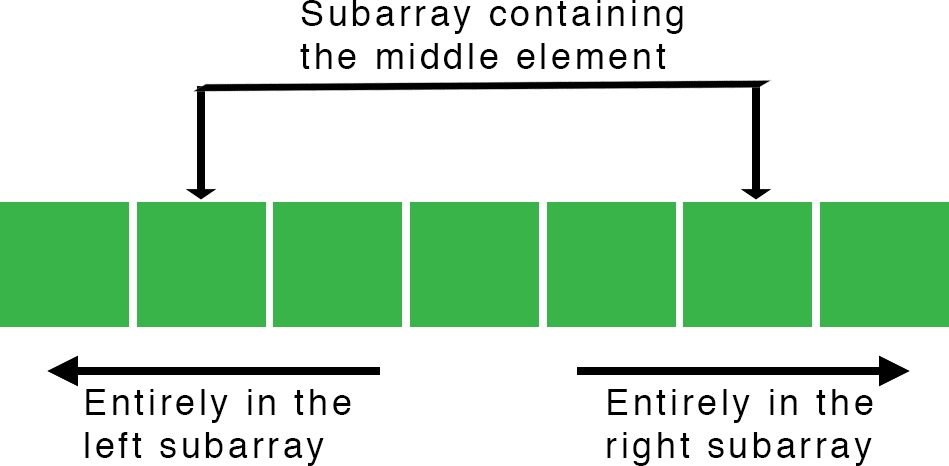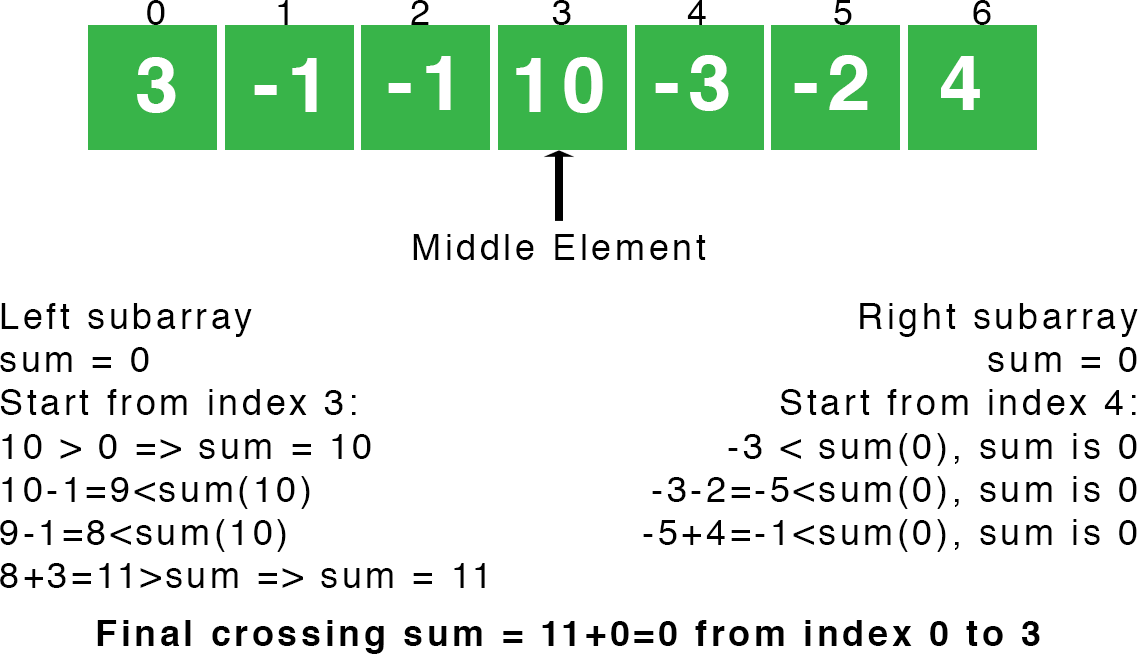# Maximum Subarray Sum Using Divide and Conquer

Nov. 13, 2018 64650

Consider visiting the divide and conquer post for the basics of divide and conquer.

The problem of maximum subarray sum is basically finding the part of an array whose elements has the largest sum. If all the elements in an array are positive then it is easy, find the sum of all the elements of the array and it has the largest sum over any other subarrays you can make out from that array.But the problem gets more interesting when some of the elements are negative then the subarray whose sum of the elements is largest over the sum of the elements of any other subarrays of that element can lie anywhere in the array.## Brute Force vs Divide and Conquer

The Brute Force technique to solve the problem is simple. Just iterate through every element of the array and check the sum of all the subarrays that can be made starting from that element i.e., check all the subarrays and this can be done in nC2 ways i.e., choosing two different elements of the array to make a subarray.

Thus, the brute force technique is of Θ(n2) time. However,  we can solve this in Θ(nlog(n)) time using divide and conquer.

## Coding Up Maximum Subarray Sum Using Divide and Conquer

As we know that the divide and conquer solves a problem by breaking into subproblems, so let's first break an array into two parts. Now, the subarray with maximum sum can either lie entirely in the left subarray or entirely in the right subarray or in a subarray consisting both i.e., crossing the middle element.The first two cases where the subarray is entirely on right or on the left are actually the smaller instances of the original problem. So, we can solve them recursively by calling the function to calculate the maximum sum subarray on both the parts.

max_sum_subarray(array, low, high)
{
if (high == low) // only one element in an array
{
return array[high]
}
mid = (high+low)/2

max_sum_subarray(array, low, mid)
max_sum_subarray(array, mid+1, high)
}


We are making max_sum_subarray is a function to calculate the maximum sum of the subarray in an array. Here, we are calling the function max_sum_subarray for both the left and the right subarrays i.e., recursively checking the left and the right subarrays for the maximum sum subarray.

Now, we have to handle the third case i.e., when the subarray with the maximum sum contains both the right and the left subarrays (containing the middle element). At a glance, this could look like a smaller instance of the original problem also but it is not because it contains a restriction that the subarray must contain the middle element and thus makes our problem much more narrow and less time taking.

So, we will now make a function called max_crossing_subarray to calculate the maximum sum of the subarray crossing the middle element and then call it inside the max_sum_subarray function.

max_sum_subarray(array, low, high)
{
if (high == low) // only one element in an array
{
return array[high];
}

mid = (high+low)/2;

maximum_sum_left_subarray = max_sum_subarray(array, low, mid)
maximum_sum_right_subarray = max_sum_subarray(array, mid+1, high)
maximum_sum_crossing_subarray = max_crossing_subarray(array, low, mid, high);

// returning the maximum among the above three numbers
return maximum(maximum_sum_left_subarray, maximum_sum_right_subarray, maximum_sum_crossing_subarray);
}


Here, we are covering all three cases mentioned above to and then just returning the maximum of these three. Now, let's write the max_crossing_subarray function.

The max_crossing_subarray function is simple, we just have to iterate over the right and the left sides of the middle element and find the maximum sum.max_crossing_subarray(int ar[], int low, int mid, int high)
{

left_sum = -infinity
sum = 0
for (i=mid downto low)
{
sum = sum+ar[i]
if (sum>left_sum)
left_sum = sum
}

right_sum = -infinity;
sum = 0

for (i=mid+1 to high)
{
sum=sum+ar[i]
if (sum>right_sum)
right_sum = sum
}

return (left_sum+right_sum)
}


Here, our first loop is iterating from the middle element to the lowest element of the left subarray to find the maximum sum and similarly the second loop is iterating from the middle+1 element to the highest element of the subarray to calculate the maximum sum of the subarray on the right side. And finally, we are returning summing both of them and returning the sum which is calculated from the subarray crossing the middle element.

### C

#include <stdio.h>

// function to return maximum number among three numbers
int maximum(int a, int b, int c)
{
if (a>=b && a>=c)
return a;
else if (b>=a && b>=c)
return b;
return c;
}

// function to find maximum sum of subarray crossing the middle element
int max_crossing_subarray(int ar[], int low, int mid, int high)
{
/*
Initial left_sum should be -infinity.
One can also use INT_MIN from limits.h
*/
int left_sum = -1000000;
int sum = 0;
int i;

/*
iterating from middle
element to the lowest element
to find the maximum sum of the left
subarray containing the middle
element also.
*/
for (i=mid; i>=low; i--)
{
sum = sum+ar[i];
if (sum>left_sum)
left_sum = sum;
}

/*
Similarly, finding the maximum
sum of right subarray containing
the adjacent right element to the
middle element.
*/
int right_sum = -1000000;
sum = 0;

for (i=mid+1; i<=high; i++)
{
sum=sum+ar[i];
if (sum>right_sum)
right_sum = sum;
}

/*
returning the maximum sum of the subarray
containing the middle element.
*/
return (left_sum+right_sum);
}

// function to calculate the maximum subarray sum
int max_sum_subarray(int ar[], int low, int high)
{
if (high == low) // only one element in an array
{
return ar[high];
}

// middle element of the array
int mid = (high+low)/2;

// maximum sum in the left subarray
int maximum_sum_left_subarray = max_sum_subarray(ar, low, mid);
// maximum sum in the right subarray
int maximum_sum_right_subarray = max_sum_subarray(ar, mid+1, high);
// maximum sum in the array containing the middle element
int maximum_sum_crossing_subarray = max_crossing_subarray(ar, low, mid, high);

// returning the maximum among the above three numbers
return maximum(maximum_sum_left_subarray, maximum_sum_right_subarray, maximum_sum_crossing_subarray);
}

int main()
{
int a[] = {3, -1, -1, 10, -3, -2, 4};
printf("%d\n", max_sum_subarray(a, 0, 6));
return 0;
}


### Python

# function to return maximum number among three numbers
def maximum(a, b, c):
if (a>=b and a>=c):
return a
elif (b>=a and b>=c):
return b
return c

# function to find maximum sum of subarray crossing the middle element
def max_crossing_subarray(ar, low, mid, high):
'''
Initial left_sum should be -infinity.
'''
left_sum = -1000000
sum = 0

'''
iterating from middle
element to the lowest element
to find the maximum sum of the left
subarray containing the middle
element also.
'''
for i in range(mid, low-1, -1):
sum = sum+ar[i]
if (sum>left_sum):
left_sum = sum

'''
Similarly, finding the maximum
sum of right subarray containing
the adjacent right element to the
middle element.
'''
right_sum = -1000000
sum = 0

for i in range(mid+1, high+1):
sum = sum+ar[i]
if (sum>right_sum):
right_sum = sum

'''
returning the maximum sum of the subarray
containing the middle element.
'''
return left_sum+right_sum

# function to calculate the maximum subarray sum
def max_sum_subarray(ar, low, high):
if (high == low): # only one element in an array
return ar[high]

# middle element of the array
mid = (high+low)//2

# maximum sum in the left subarray
maximum_sum_left_subarray = max_sum_subarray(ar, low, mid)
# maximum sum in the right subarray
maximum_sum_right_subarray = max_sum_subarray(ar, mid+1, high)
# maximum sum in the array containing the middle element
maximum_sum_crossing_subarray = max_crossing_subarray(ar, low, mid, high)

# returning the maximum among the above three numbers
return maximum(maximum_sum_left_subarray, maximum_sum_right_subarray, maximum_sum_crossing_subarray)

a = [3, -1, -1, 10, -3, -2, 4]
print(max_sum_subarray(a, 0, 6))


### Java

class MaxSubarray {

// function to return maximum number among three numbers
static int maximum(int a, int b, int c)
{
if (a>=b && a>=c)
return a;
else if (b>=a && b>=c)
return b;
return c;
}

// function to find maximum sum of subarray crossing the middle element
static int maxCrossingSubarray(int ar[], int low, int mid, int high)
{
/*
Initial leftSum should be -infinity.
*/
int leftSum = Integer.MIN_VALUE;
int sum = 0;
int i;

/*
iterating from middle
element to the lowest element
to find the maximum sum of the left
subarray containing the middle
element also.
*/
for (i=mid; i>=low; i--)
{
sum = sum+ar[i];
if (sum>leftSum)
leftSum = sum;
}

/*
Similarly, finding the maximum
sum of right subarray containing
the adjacent right element to the
middle element.
*/
int rightSum = Integer.MIN_VALUE;
sum = 0;

for (i=mid+1; i<=high; i++)
{
sum=sum+ar[i];
if (sum>rightSum)
rightSum = sum;
}

/*
returning the maximum sum of the subarray
containing the middle element.
*/
return (leftSum+rightSum);
}

// function to calculate the maximum subarray sum
static int maxSumSubarray(int ar[], int low, int high)
{
if (high == low) // only one element in an array
{
return ar[high];
}

// middle element of the array
int mid = (high+low)/2;

// maximum sum in the left subarray
int maximumSumLeftSubarray = maxSumSubarray(ar, low, mid);
// maximum sum in the right subarray
int maximumSumRightSubarray = maxSumSubarray(ar, mid+1, high);
// maximum sum in the array containing the middle element
int maximumSumCrossingSubarray = maxCrossingSubarray(ar, low, mid, high);

// returning the maximum among the above three numbers
return maximum(maximumSumLeftSubarray, maximumSumRightSubarray, maximumSumCrossingSubarray);
}

public static void main(String[] args) {
int a[] = {3, -1, -1, 10, -3, -2, 4};
System.out.println(maxSumSubarray(a, 0, 6));
}
}


Liked the post?
Developer and founder of CodesDope.
0 COMMENT